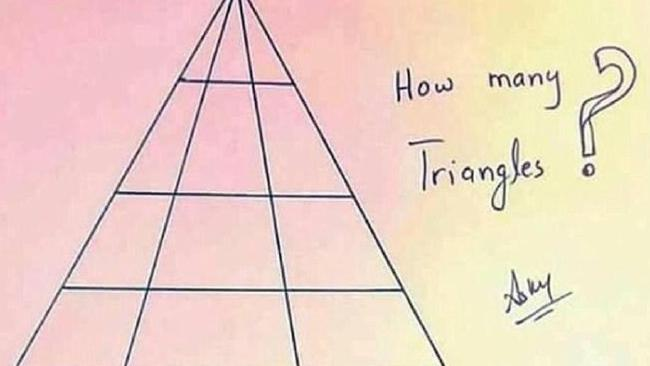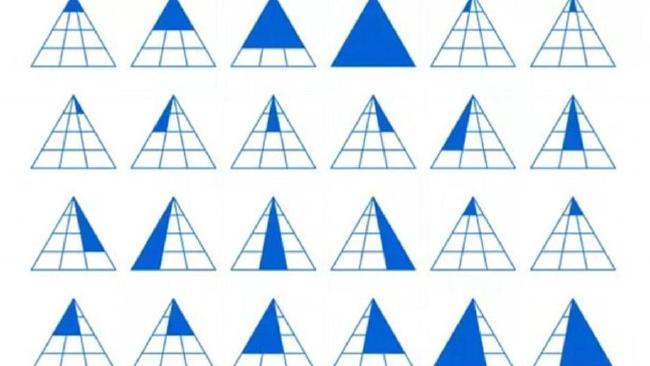Can you figure out how many triangles are hidden within this illustration? Picture: Kumar Ankit/Quora

# How many triangles are in this picture?

A BRAINTEASER that asks users to calculate the number of triangles in an image is leaving the web baffled.

The simple illustration, which was posted by Kumar Ankit on Quora, has now been shared thousands of times on Facebook after leaving viewers scratching their heads, reports The Sun.

Those attempting to solve the conundrum have been giving an array of different answers - ranging from 4 to a sizeable 44.

But can you figure out the correct answer?

While hordes of people have left their calculations in the comments section, no one can seem to agree on the answer.

The solution is actually 25 - there are 24 smaller triangles within the main triangle, as well as one in the signature.Martin Silvertant, a mathematician, has created a handy chart to explain how he reached the answer. Picture: Martin SilvertantSource:Supplied

There are six in the top row - three individuals triangles, two pairs, and one comprising of all three.

Then there's six in second row, six in the third and six in the fourth row using the same approach.

There's also a triangle in the signature written underneath the question - in the "A".

Martin Silvertant, a mathematician, has created a handy chart to explain how he reached the answer.

But some shrewd users have also pointed out that you could include the word "triangles" in the answer - to reach 26.

Did you solve it correctly? Let us know in the comments below.